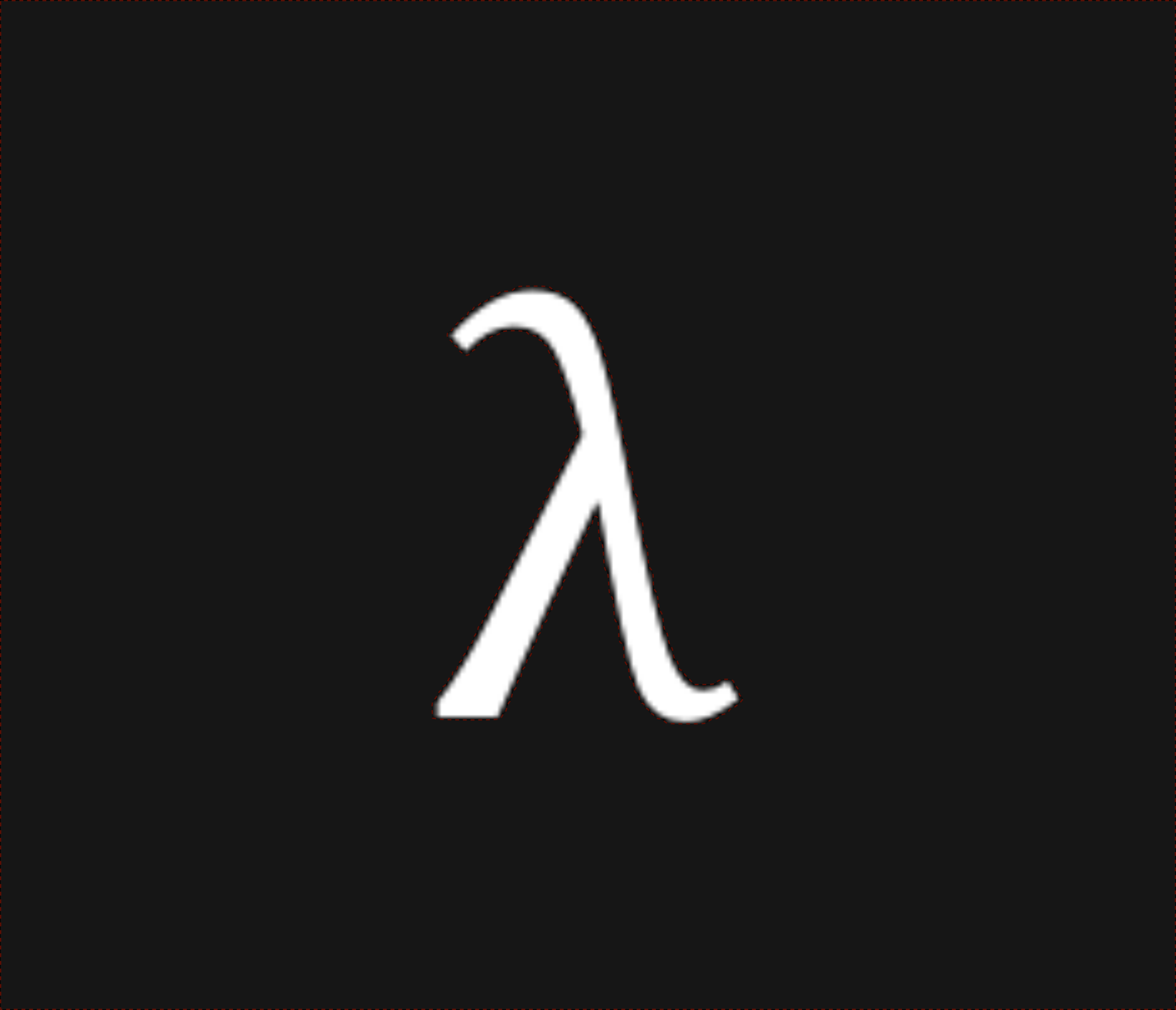nixpulvis

## (S)MPC & An Uncertain Calculus 622 words written by Nathan Lilienthal

Abstract: Towards a minimal language for multi-party communication and computations. I (we?) define two new languages: $$\lambda_\div$$, a language for probabilistic computation, and $$\lambda_o$$ a language for multi-party secure computation.

$$\lambda_o$$ is an extension of $$\lambda_\div$$, however it doesn’t need to export the idea of a $$\div$$ flipping primitive.

### Secure Two Party Communication (S2PC)

Generally, both parties will have an input to a function $$\lambda_o$$, here we’ll call $$\color{blue} A$$’s input $$\color{blue} x$$ and $$\color{red} B$$’s input $$\color{red} y$$. Before either party can pass their inputs to $$\lambda_o$$ they must create an authentication context with $$\bullet \ e$$.

$(\lambda_o \ \color{blue}{x} \ \color{red}{y} \ . e) \ \color{blue}{\bullet}a \ \color{red}{\bullet}b \rightarrow e[a/\color{blue} x,b/\color{red} y]$

We have up to three possible implementations for $$e$$: $$e$$, $$\color{blue}{\bullet \ e}$$ and $$\color{red}{\bullet \ e}$$. This allows for each party to perform arbitrary computations on the oblivious data, or to fix the garbling up front so no one party controls it. For example here the expression $$e$$ was garbled by $$\color{blue}A$$.

$(\lambda_o \ \color{blue}{x} \ \color{red}{y} \ . \color{blue}{\bullet}\ e) \ \color{blue}{\bullet}a \ \color{red}{\bullet}b \rightarrow \color{blue}{\bullet \ e[\color{black}a/\color{blue}x,\color{black}b/\color{red}y]}$

Traditional function notation can be colored to indicate the same notion.

$\color{blue}{\bullet} \ f \ \color{blue}x \ \color{red}y = \color{blue}{f(x,\color{red} y)} \\ \color{red}{\bullet} g \ \color{blue}x \ \color{red}y = \color{red}{g(\color{blue}x,y)}$

We see that each party, $$\color{blue} A$$ and $$\color{red} B$$, have unique functions, $$\color{blue} f$$ and $$\color{red} g$$ respectively. However it is also possible to define $$e = \color{blue}{f} \cup \color{red}{g}$$. These functions are oblivious to the other party’s data but follow consistent use.

One example pair of $$\color{blue} f$$ and $$\color{red} g$$ functions is a classic problem of Oblivious Transfer (OT). In this case, $$\color{blue} A$$ wants to access a specific element from $$\color{red} B$$’s database, without $$\color{red} B$$ knowing what element was retrieved. For this example, we’ll use a database projection function $$\color{red}{p}$$ (oblivious to anything else about the database), which takes an oblivious index $$\color{blue} i$$.

$\Gamma \vdash \lambda_o \ \color{blue}{i} \ \color{red}{p} \ . \color{red}{p} \ \color{blue}{i} : \sigma_o \rightarrow (\sigma_o \rightarrow \tau_o) \rightarrow \tau_o \\ \cong \\ \Gamma \vdash \lambda_o \ \color{blue}{i} \ \color{red}{p} \ . \color{red}{p} \ \color{blue}{i} : \sigma_o \times (\sigma_o \times \tau_o) \rightarrow \tau_o$

Or as a solution to Yao’s Millionare Problem:

$\Gamma, >: \mathbb{Z} \times \mathbb{Z} \rightarrow \{0,1\} \ \vdash (\lambda_o \ \color{blue}a \ \color{red}b \ . \color{blue}a > \color{red}b)$

It’s worth noting that the $$>$$ operator in the figure above is written in black because it’s “baked in” to the protocol.

### S3PC

This notion expands naturally for a third member of the computation, Carrol ($$\color{green} C$$). Similar to the S2PC case above, we can define a $$\lambda_o$$, which now takes three arguments and tells each member what position in line they are in a group.

$\lambda_o \ \color{blue}{x} \ \color{red}{y} \ \color{green}{z} \ . \\ \color{blue}{place(x, \left\{x,\color{red}y,\color{green}z\}\right)} \\ \color{red}{place(y, \left\{\color{blue}x,y,\color{green}z\}\right)} \\ \color{green}{place(z, \left\{\color{blue}x,\color{red}y,z\}\right)}$

### $$\lambda_\div$$ the Uncertainty Calculus

$\div \ a \\ 50\% \rightarrow a \\ 50\% \rightarrow \_$ $\div \div a \\ 25\% \rightarrow a \\ 75\% \rightarrow \_$ $\div \ a \ b \\ 50\% \rightarrow a \ b \\ 50\% \rightarrow b \ a$ $a \div b \\ \div \div \ a \ b \\ 50\% \rightarrow a \ b \\ 50\% \rightarrow b \ a$

Below the order of operations $$f \ g$$ is certain, while the order of $$a \ b$$ is uncertain.

$\lambda f \ g \ a \ b . f \ g \div a \ b \\$

Full expressions can be wrapped and made uncertain.

$\lambda \_ . \div \ (\lambda a \ b . a \ b) \ (\lambda a \ b . b \ a)$

Much more work is clearly needed on this idea…